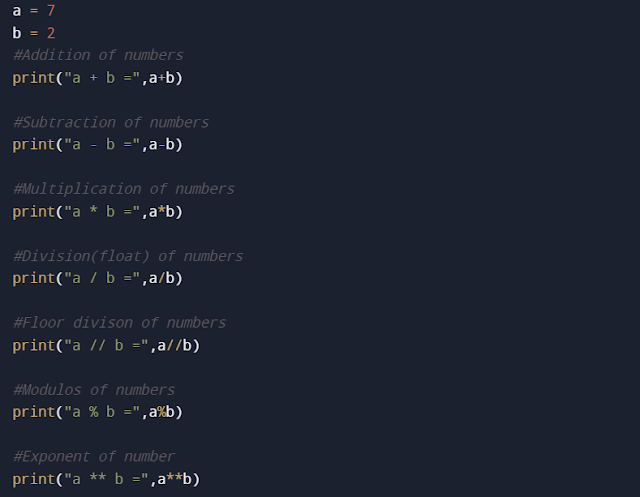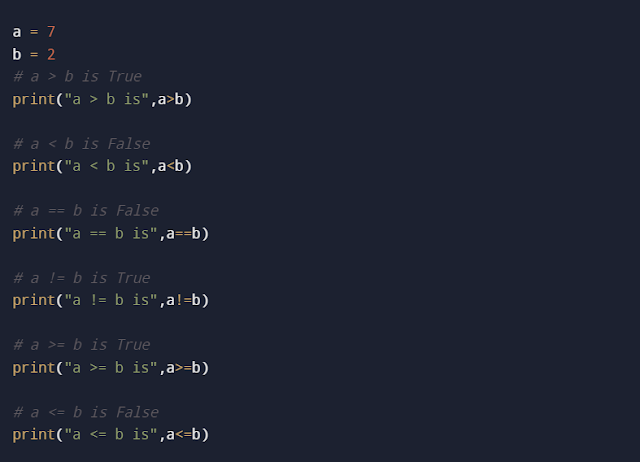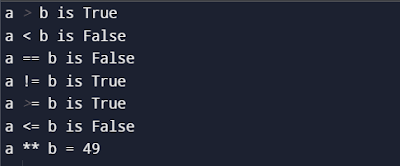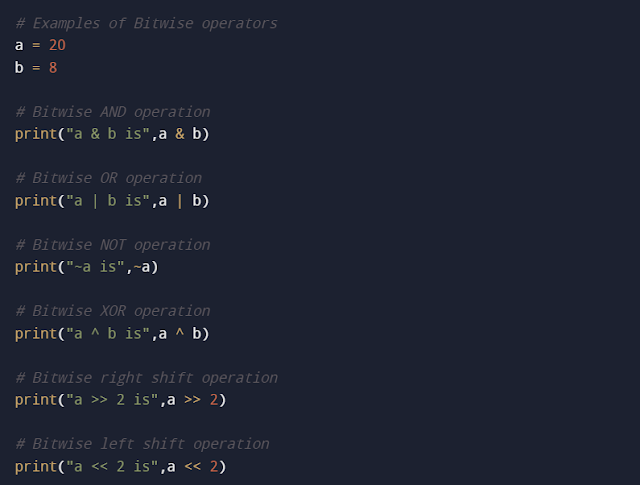## Pages

### Python Operators

Python Operators:
• Operators are special symbols that used to perform operations on the variables and the values.
• Consider an expression 10 + 20 = 30. Here, + is an operator that performs addition. 10 and 20 are called operands and 30 is the output of the operation.
• In general, the operand is a variable or a value that the operator operates on.
• Most commonly used operator groups in python language are listed below
• Arithmetic Operators
• Comparison/Relational Operators
• Logical Operators
• Assignment Operators
• Bitwise Operators
• Identity Operators
• Membership Operators
• Let's have a look at each operator with their syntax.
Arithmetic Operators:
• Arithmetic operators are used with numeric values to perform mathematical operations like addition, subtraction, multiplication, division, etc.,
 Operator Name Description Syntax Example + Addition Add two operands a + b - Subtraction Subtract right operand from the left operand a - b * Multiplication Multiply two operands a * b / Division Divide left operand by right operand (result – float) a / b % Modulus Returns the remainder of the division of left operand by the right operand a % b // Floor Division Returns Quotient of the division of left operand by right operand (result – the whole number) a // b ** Exponentiation Exponent – Left operand raised to the power of right operand a ** b

• Example: Arithmetic operators in PythonArithmetic operators PythonOutput

Comparison/Relational Operators:
• Comparison operators are used to compare two values and returns either True or False based on the condition.
 Operator Name Description Syntax Example == Equal Returns True – If both operands are equal a == b != Not Equal Returns True – If both operands are not equal a != b > Greater than Returns True – If the left operand is greater than right operand a > b < Less than Returns True – If the left operand is less than the right operand a < b >= Greater than or equal to Returns True – If the left operand is greater than or equal to the right operand a >= b <= Less than or equal to Returns True – If the left operand is lesser than or equal to the right operand a <= b

• Example: Comparison operators in PythonComparison operators PythonOutput
Logical Operators:
• Logical operators are used to combine conditional statements or variables or values using Logical AND, Logical OR, and Logical Not.
 Operator Name Description Syntax Example and Logical AND Returns True – If both operands are true a and b or Logical OR Returns True – If either of the operands is true a or b not Logical NOT Returns True – If the operand is false not a

• Example: Logical operators in PythonLogical Operators PythonOutput
Assignment Operators:
• Assignment operators are used to assign values to variables.
 Operator Name Description Syntax Example = Assign Assign the value of right operand to left operand c = a + b += Add and Assign Add right operand with left operand and then assign to left operand a+=b --> a = a+b -= Subtract and Assign Subtract right operand from left operand and then assign to left operand a-=b --> a = a-b *= Multiply and Assign Multiply right operand with left operand and then assign to left operand a*=b --> a = a*b /= Divide and Assign Divide left operand with right operand and then assign to left operand a/=b --> a = a/b %= Modulus and Assign Takes remainder using left and right operand and then assign to left operand a%=b --> a = a%b //= Floor Division and Assign Divide left operand with right operand and then assign floor value to left operand a//=b --> a = a//b **= Exponent and Assign Finds exponent value using operands and then assign value to left operand a**=b --> a = a**b &= Bitwise AND and Assign Perform Bitwise AND on operands and then assign value to left operand a&=b --> a = a&b |= Bitwise OR and Assign Perform Bitwise OR on operands and then assign value to left operand a|=b --> a = a|b ^= Bitwise XOR and Assign Perform Bitwise XOR on operands and then assign value to left operand a^=b --> a = a^b >>= Bitwise right shift and Assign Perform Bitwise right shift on operands and then assign value to left operand a>>=b --> a = a>>b <<= Bitwise left shift and Assign Perform Bitwise left shift on operands and then assign value to left operand a<<=b --> a = a<

Bitwise Operators:
• Bitwise operators work on bits and perform bit by bit operation.
• For example, 4 is 100 in binary, and 7 is 111.
 Operator Name Description Syntax Example & Bitwise AND The operator sets bit result to 1 if both operand bits are 1 x & y | Bitwise OR The operator sets bit result to 1 if one of the two operand bits is 1 x | y ~ Bitwise NOT Inverts all the bits ~ x ^ Bitwise XOR The operator sets bit result to 1 if only one of the two operand bits is 1, not both x ^ y >> Bitwise right shift Left operand value is moved right by the number of bits specified by the right operand x >> << Bitwise left shift Left operand value is moved left by the number of bits specified by the right operand x <<

• Example: Bitwise operators in PythonBitwise operator PythonOutput
Identity Operators:
• Identity operators are used to compare the memory location of the objects.
• If two variables are equal in value, it doesn't imply that they are identical.
 Operator Description Syntax Example is Returns True – If both variables refer to the same object x is y is not Returns True – If both variables do not refer to the same object x is not y

• Example: Identity operators in PythonIdentity Operator PythonOutput
Membership Operators:
• Membership operators are used to test whether a value or a variable is found in a collection sequence ( list, string, tuple, set, and dictionary).
• In a dictionary, we can check only the presence of the key, not the value.

 Operator Description Syntax Example In Returns True – If value/variable is found in the specified sequence. x in y not in Returns True – If value/variable is not found in the specified sequence. x not in y

• Example: Membership operators in PythonMembership Operator PythonOutput